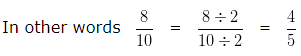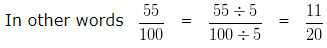# Convert Decimal to Fraction

To Convert Decimal to Fraction we have to follow the following steps.

Step 1: Write the given Decimal without the Decimal point as the numerator of the fraction.

Step 2: In the Denominator, write 1 followed by as many zeroes, as there are Decimal places in the given Decimal.

Step 3: Convert the fraction into simplest form.

### Convert Decimal to Fraction

Convert Decimal to Fraction – Example 1

Convert 0.8 into a fraction in its Simplest form?

Solution

Step 1: Write the given Decimal without the Decimal point as the numerator of the fraction 8

Step 2: Write 1 followed by as many zeroes, as there are Decimal places in the given Decimal 10 (Since there was 1 decimal place in the given number, we have added 1 zero at the end of 1 )

= 8/10

Step 3: Convert the fraction 8/10 into simplest form

HCF of 8 and 10 is 2

To simplify this we have to divide both the numerator and denominator of the fraction by their HCF i.e,

8 and 10 by 2Hence, 0.8 = 4/5

Convert Decimal to Fraction – Example 2

Convert 0.55 into a fraction in its Simplest form?

Solution

Step 1: Write the given Decimal without the Decimal point as the numerator of the fraction 55

Step 2: Write 1 followed by as many zeroes, as there are Decimal places in the given Decimal 100 (Since there was 2 decimal place in the given number, we have added 2 zero at the end of 1 )

= 55/100

Step 3: Convert the fraction 55/100 into simplest form

HCF of 55 and 100 is 5

To simplify this we have to divide both the numerator and denominator of the fraction by their HCF i.e,

55 and 100 by 5Hence, 0.55 = 11/20

Click here for Class 6 Chapterwise Explanations